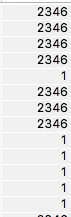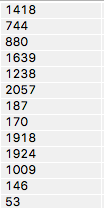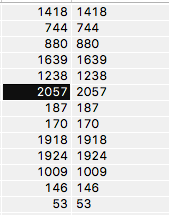# Rnd( function giving only two values

In Panorama 6, this statement in a numeric field:

``````formulafill int(1+2345*rnd())
``````

gives this result:but, in a text field, this:

``````formulafill str(int(1+2345*rnd())) gives:
``````and I can use the `val(` function to put the correct values in the original numeric field:This happens on both my iMac and my MacBook Pro. What’s going on?

More specifically, you mean in an integer numeric field. If you use a floating point field, it will work correctly.

When a Panorama 6 function or operator has operands with different data types, it converts both of them to the final field type, then performs the operation. In this case

``````2345
``````

is an integer, and

``````rnd(
``````

is a floating point number from 0 to 1. Since an integer and a floating point number are different types, Panorama converts them to the type of the integer if the result is going to be an integer. Then it does the multiply.

If you convert the output of rnd( to an integer, you will get 0 or 1, so that is what you are seeing.

If the final result is a text field, Panorama treats that the same as a floating point field, so in that case 2345 is converted to a floating point number, and rnd( is already a floating point number. So it works great.

Why does Panorama 6 do this in such a dumb way? Remember, this program was originally started in 1986, when floating point operations were very, very slow. So converting integers and fixed point values to floating point, then back again, would be quite slow.

You can make your formula work in an integer field by explicitly converting everything to float(, leaving Panorama with no freedom to decide how to do the conversion.

``````formulafill int(float(1)+float(2345)*rnd())
``````

Panorama X doesn’t have this problem. If operands don’t match, they are always converted to floating point. And the fixed point numeric types don’t even exist any more. Floating point is super fast now, so that isn’t a problem, and you no longer have to worry about underflows and overflows any more.

Understood. The Panorama 6 Programming Reference doesn’t make that point at all, in fact it gives erroneous examples but that’s all water under the bridge now.

In fact, the Panorama X Help entry is even less helpful.

Page 61 of the Formulas & Programming book does discuss this topic, in the section Overflow/Underflow Problems.

First of all, what entry are you referring to?

Secondly, I believe there’s no need for any explanation in the Panorama X help, because this issue doesn’t exist, as I explained above. Your original formula works fine on an integer field in Panorama X. In fact, the int( function is not required.